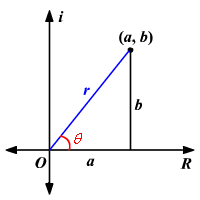# Polar Form of a Complex Number

The polar form of a complex number is another way to represent a complex number. The form $z=a+bi$ is called the rectangular coordinate form of a complex number.The horizontal axis is the real axis and the vertical axis is the imaginary axis. We find the real and complex components in terms of $r$ and $\theta$ where $r$ is the length of the vector and $\theta$ is the angle made with the real axis.

From Pythagorean Theorem :

${r}^{2}={a}^{2}+{b}^{2}$

By using the basic trigonometric ratios :

$\mathrm{cos}\theta =\frac{a}{r}$ and $\mathrm{sin}\theta =\frac{b}{r}$ .

Multiplying each side by $r$ :

The rectangular form of a complex number is given by

$z=a+bi$ .

Substitute the values of $a$ and $b$ .

$\begin{array}{l}z={a}+{b}i\\ \text{\hspace{0.17em}}\text{\hspace{0.17em}}\text{\hspace{0.17em}}\text{\hspace{0.17em}}={r}{\mathrm{cos}}{\theta }+\left({r}{\mathrm{sin}}{\theta }\right)i\\ \text{\hspace{0.17em}}\text{\hspace{0.17em}}\text{\hspace{0.17em}}\text{\hspace{0.17em}}=r\left(\mathrm{cos}\theta +i\mathrm{sin}\theta \right)\end{array}$

In the case of a complex number, $r$ represents the absolute value or modulus and the angle $\theta$ is called the argument of the complex number.

This can be summarized as follows:

The polar form of a complex number $z=a+bi$ is $z=r\left(\mathrm{cos}\theta +i\mathrm{sin}\theta \right)$ , where $r=|z|=\sqrt{{a}^{2}+{b}^{2}}$ , , and $\theta ={\mathrm{tan}}^{-1}\left(\frac{b}{a}\right)$ for $a>0$ and $\theta ={\mathrm{tan}}^{-1}\left(\frac{b}{a}\right)+\pi$ or $\theta ={\mathrm{tan}}^{-1}\left(\frac{b}{a}\right)+180°$ for $a<0$ .

Example:

Express the complex number in polar form.

$5+2i$

The polar form of a complex number $z=a+bi$ is $z=r\left(\mathrm{cos}\theta +i\mathrm{sin}\theta \right)$ .

So, first find the absolute value of $r$ .

$\begin{array}{l}r=|z|=\sqrt{{a}^{2}+{b}^{2}}\\ \text{\hspace{0.17em}}\text{\hspace{0.17em}}\text{\hspace{0.17em}}\text{\hspace{0.17em}}=\sqrt{{5}^{2}+{2}^{2}}\\ \text{\hspace{0.17em}}\text{\hspace{0.17em}}\text{\hspace{0.17em}}\text{\hspace{0.17em}}=\sqrt{25+4}\\ \text{\hspace{0.17em}}\text{\hspace{0.17em}}\text{\hspace{0.17em}}\text{\hspace{0.17em}}=\sqrt{29}\\ \text{\hspace{0.17em}}\text{\hspace{0.17em}}\text{\hspace{0.17em}}\text{\hspace{0.17em}}\text{\hspace{0.17em}}\approx 5.39\end{array}$

Now find the argument $\theta$ .

Since $a>0$ , use the formula $\theta ={\mathrm{tan}}^{-1}\left(\frac{b}{a}\right)$ .

$\begin{array}{l}\theta ={\mathrm{tan}}^{-1}\left(\frac{2}{5}\right)\\ \text{\hspace{0.17em}}\text{\hspace{0.17em}}\text{\hspace{0.17em}}\text{\hspace{0.17em}}\approx 0.38\end{array}$

Note that here $\theta$ is measured in radians.

Therefore, the polar form of $5+2i$ is about $5.39\left(\mathrm{cos}\left(0.38\right)+i\mathrm{sin}\left(0.38\right)\right)$ .